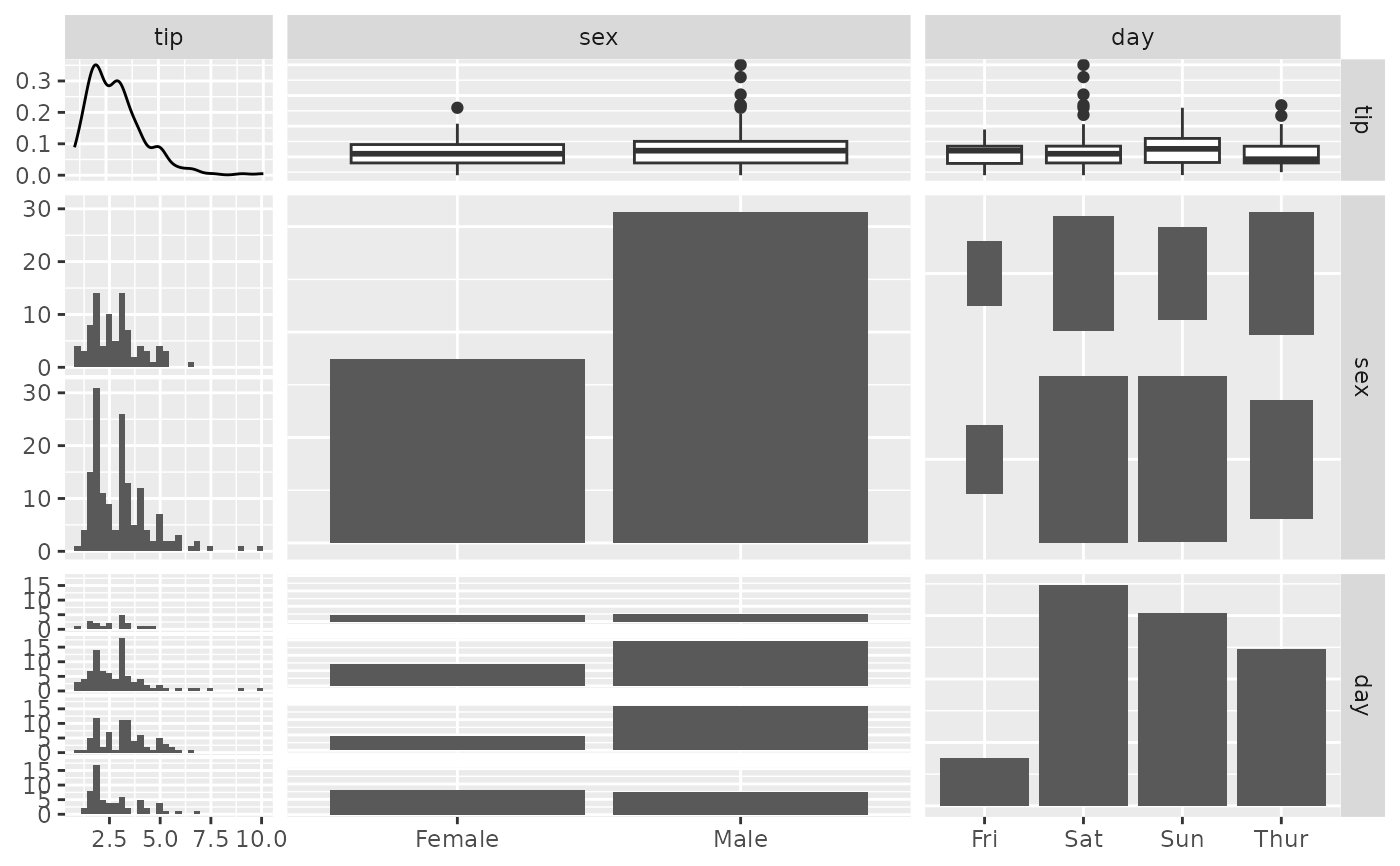Make a matrix of plots with a given data set

ggpairs(
data,
mapping = NULL,
columns = 1:ncol(data),
title = NULL,
upper = list(continuous = "cor", combo = "box_no_facet", discrete = "count", na = "na"),
lower = list(continuous = "points", combo = "facethist", discrete = "facetbar", na =
"na"),
diag = list(continuous = "densityDiag", discrete = "barDiag", na = "naDiag"),
params = NULL,
...,
xlab = NULL,
ylab = NULL,
axisLabels = c("show", "internal", "none"),
columnLabels = colnames(data[columns]),
labeller = "label_value",
switch = NULL,
showStrips = NULL,
legend = NULL,
cardinality_threshold = 15,
progress = NULL,
proportions = NULL,
legends = stop("deprecated")
)

## Arguments

data

data set using. Can have both numerical and categorical data.

mapping

aesthetic mapping (besides x and y). See aes(). If mapping is numeric, columns will be set to the mapping value and mapping will be set to NULL.

columns

which columns are used to make plots. Defaults to all columns.

title, xlab, ylab

title, x label, and y label for the graph

upper

see Details

lower

see Details

diag

see Details

params

deprecated. Please see wrap_fn_with_param_arg

...

deprecated. Please use mapping

axisLabels

either "show" to display axisLabels, "internal" for labels in the diagonal plots, or "none" for no axis labels

columnLabels

label names to be displayed. Defaults to names of columns being used.

labeller

labeller for facets. See labellers. Common values are "label_value" (default) and "label_parsed".

switch

switch parameter for facet_grid. See ggplot2::facet_grid. By default, the labels are displayed on the top and right of the plot. If "x", the top labels will be displayed to the bottom. If "y", the right-hand side labels will be displayed to the left. Can also be set to "both"

showStrips

boolean to determine if each plot's strips should be displayed. NULL will default to the top and right side plots only. TRUE or FALSE will turn all strips on or off respectively.

legend

May be the two objects described below or the default NULL value. The legend position can be moved by using ggplot2's theme element pm + theme(legend.position = "bottom")

a single numeric value

provides the location of a plot according to the display order. Such as legend = 3 in a plot matrix with 2 rows and 5 columns displayed by column will return the plot in position c(1,2)

a object from grab_legend()

a predetermined plot legend that will be displayed directly

cardinality_threshold

maximum number of levels allowed in a character / factor column. Set this value to NULL to not check factor columns. Defaults to 15

progress

NULL (default) for a progress bar in interactive sessions with more than 15 plots, TRUE for a progress bar, FALSE for no progress bar, or a function that accepts at least a plot matrix and returns a new progress::progress_bar. See ggmatrix_progress.

proportions

Value to change how much area is given for each plot. Either NULL (default), numeric value matching respective length, grid::unit object with matching respective length or "auto" for automatic relative proportions based on the number of levels for categorical variables.

legends

deprecated

## Value

ggmatrix object that if called, will print

## Details

upper and lower are lists that may contain the variables 'continuous', 'combo', 'discrete', and 'na'. Each element of the list may be a function or a string. If a string is supplied, it must be a character string representing the tail end of a ggally_NAME function. The list of current valid ggally_NAME functions is visible in a dedicated vignette.

continuous

This option is used for continuous X and Y data.

combo

This option is used for either continuous X and categorical Y data or categorical X and continuous Y data.

discrete

This option is used for categorical X and Y data.

na

This option is used when all X data is NA, all Y data is NA, or either all X or Y data is NA.

diag is a list that may only contain the variables 'continuous', 'discrete', and 'na'. Each element of the diag list is a string implementing the following options:

continuous

exactly one of ('densityDiag', 'barDiag', 'blankDiag'). This option is used for continuous X data.

discrete

exactly one of ('barDiag', 'blankDiag'). This option is used for categorical X and Y data.

na

exactly one of ('naDiag', 'blankDiag'). This option is used when all X data is NA.

If 'blank' is ever chosen as an option, then ggpairs will produce an empty plot.

If a function is supplied as an option to upper, lower, or diag, it should implement the function api of function(data, mapping, ...){#make ggplot2 plot}. If a specific function needs its parameters set, wrap(fn, param1 = val1, param2 = val2) the function with its parameters.

wrap v1_ggmatrix_theme

## Author

Barret Schloerke, Jason Crowley, Di Cook, Heike Hofmann, Hadley Wickham

## Examples

# small function to display plots only if it's interactive
p_ <- GGally::print_if_interactive

## Quick example, with and without colour
data(flea)
ggpairs(flea, columns = 2:4)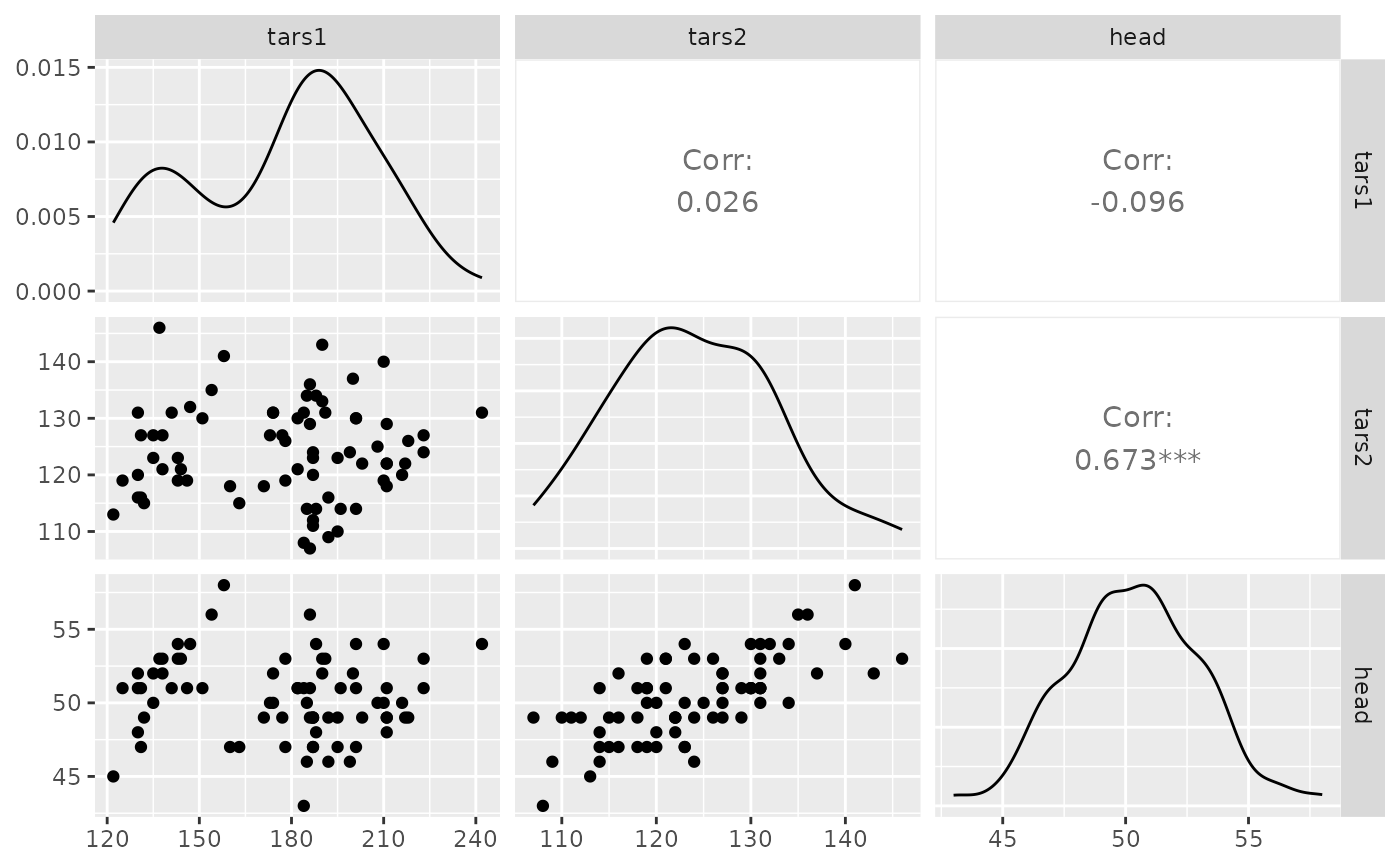pm <- ggpairs(flea, columns = 2:4, ggplot2::aes(colour = species))
p_(pm)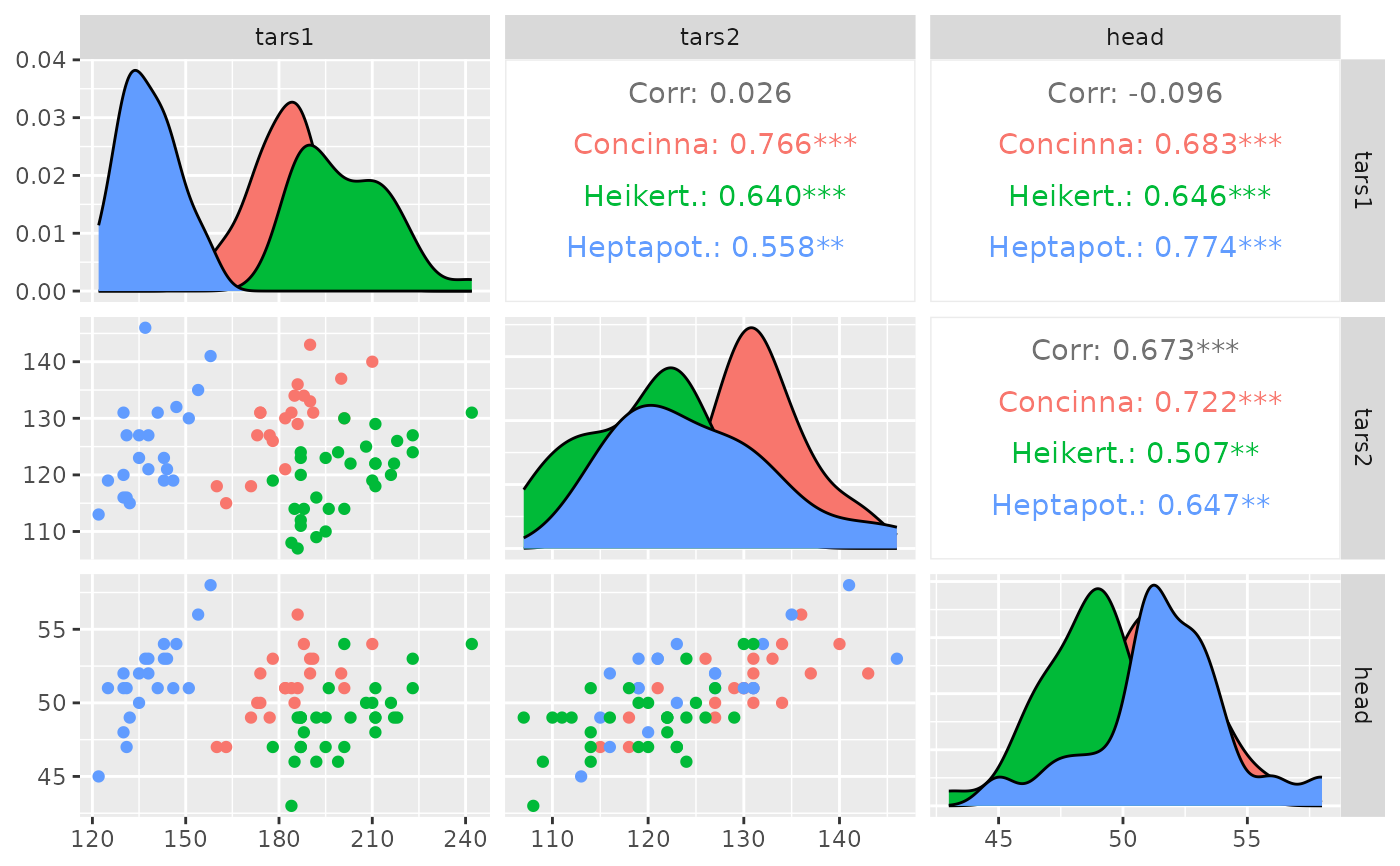# Note: colour should be categorical, else you will need to reset
# the upper triangle to use points instead of trying to compute corr

data(tips, package = "reshape")
pm <- ggpairs(tips[, 1:3])
p_(pm)
#> stat_bin() using bins = 30. Pick better value with binwidth.
#> stat_bin() using bins = 30. Pick better value with binwidth.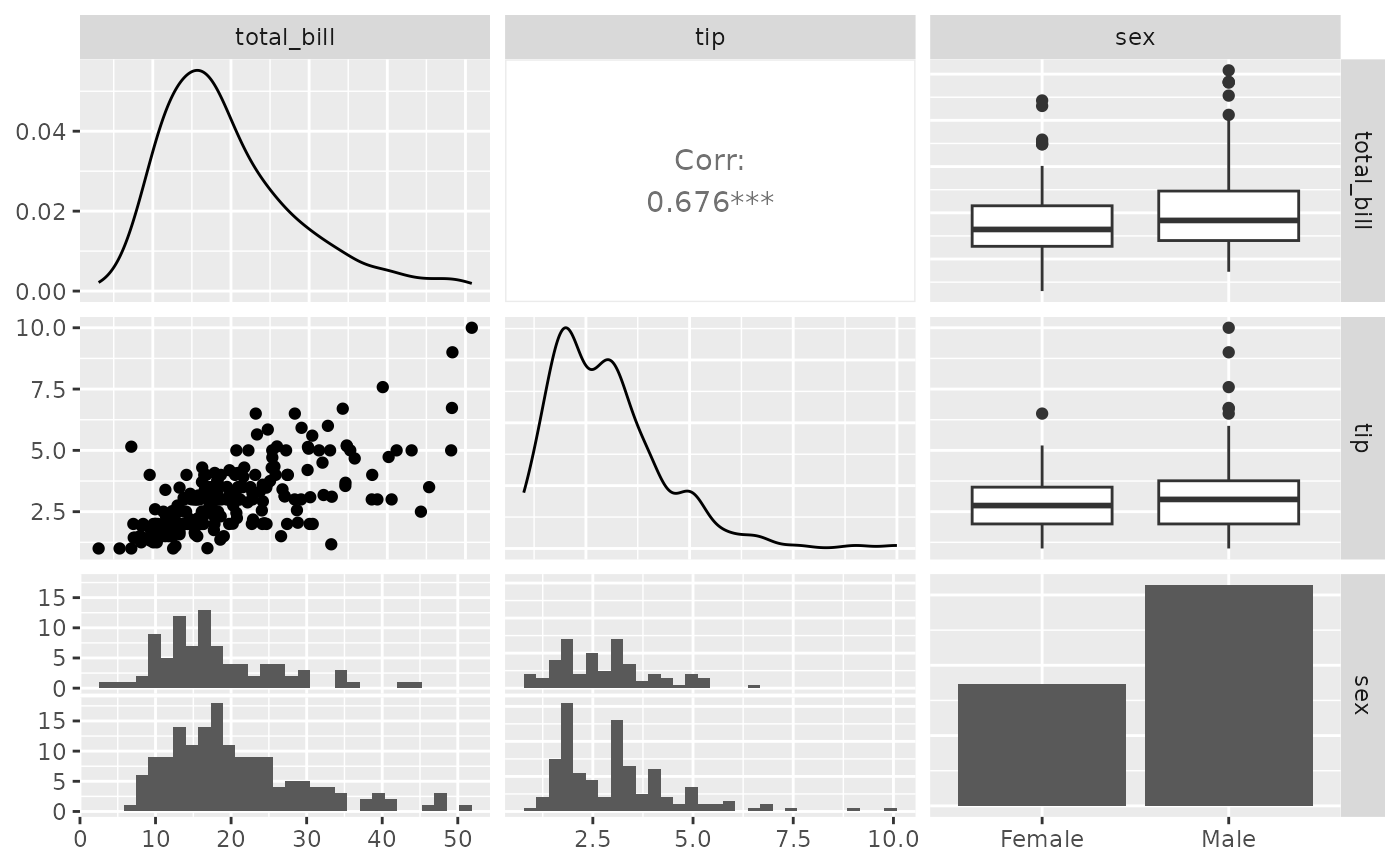pm <- ggpairs(tips, 1:3, columnLabels = c("Total Bill", "Tip", "Sex"))
p_(pm)
#> stat_bin() using bins = 30. Pick better value with binwidth.
#> stat_bin() using bins = 30. Pick better value with binwidth.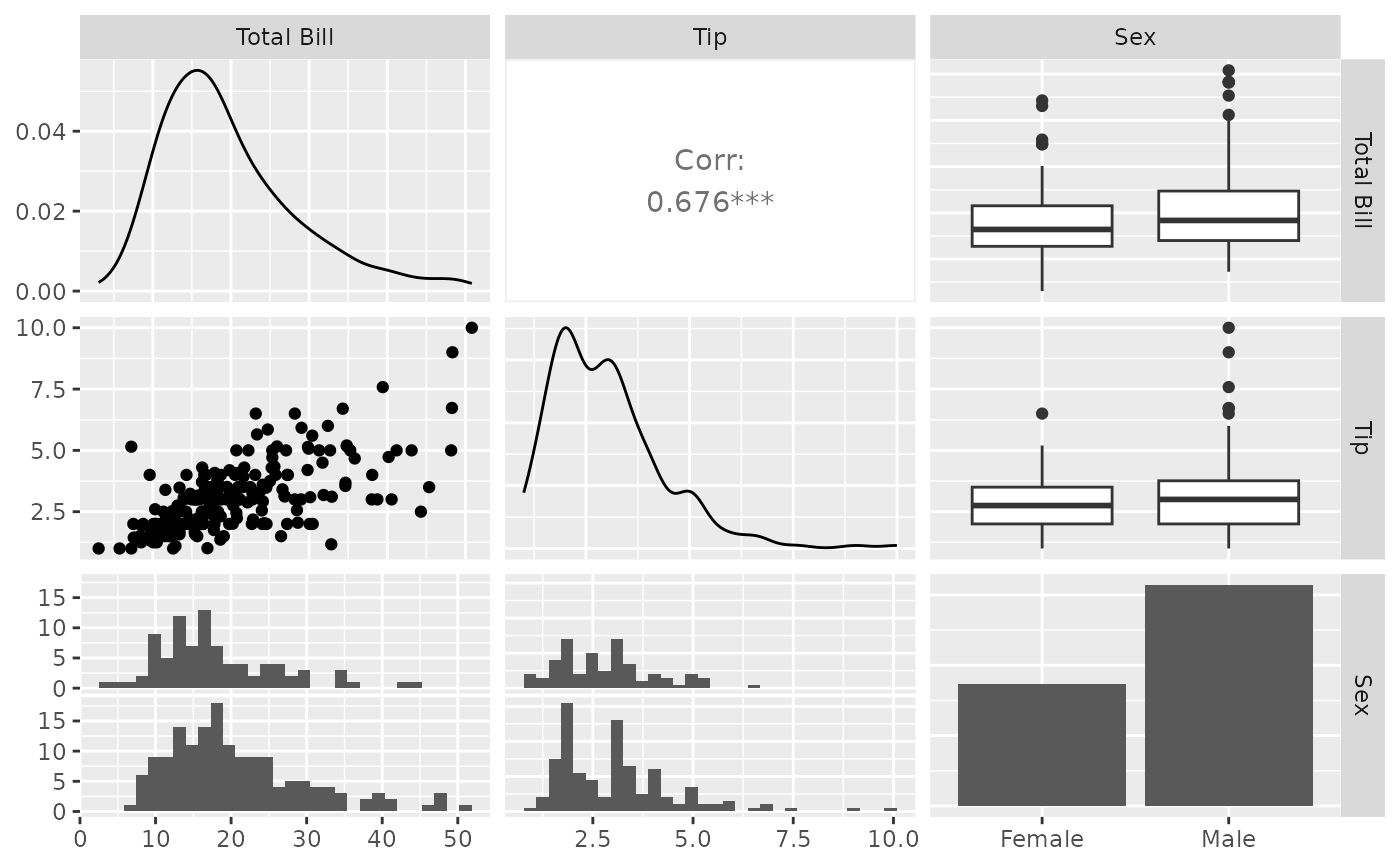pm <- ggpairs(tips, upper = "blank")
p_(pm)
#> stat_bin() using bins = 30. Pick better value with binwidth.
#> stat_bin() using bins = 30. Pick better value with binwidth.
#> stat_bin() using bins = 30. Pick better value with binwidth.
#> stat_bin() using bins = 30. Pick better value with binwidth.
#> stat_bin() using bins = 30. Pick better value with binwidth.
#> stat_bin() using bins = 30. Pick better value with binwidth.
#> stat_bin() using bins = 30. Pick better value with binwidth.
#> stat_bin() using bins = 30. Pick better value with binwidth.
#> stat_bin() using bins = 30. Pick better value with binwidth.
#> stat_bin() using bins = 30. Pick better value with binwidth.
#> stat_bin() using bins = 30. Pick better value with binwidth.
#> stat_bin() using bins = 30. Pick better value with binwidth.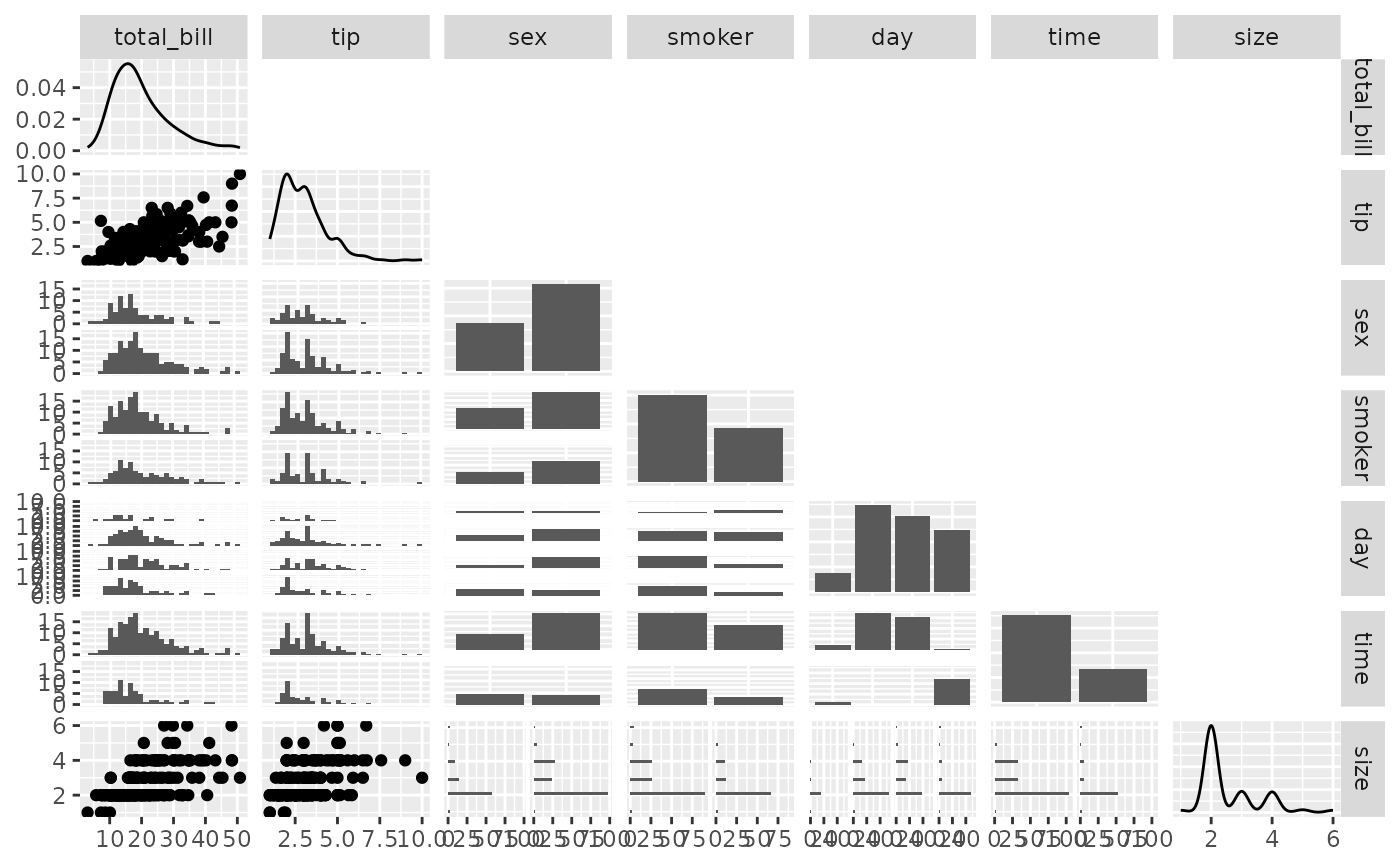## Plot Types
# Change default plot behavior
pm <- ggpairs(
tips[, c(1, 3, 4, 2)],
upper = list(continuous = "density", combo = "box_no_facet"),
lower = list(continuous = "points", combo = "dot_no_facet")
)
p_(pm)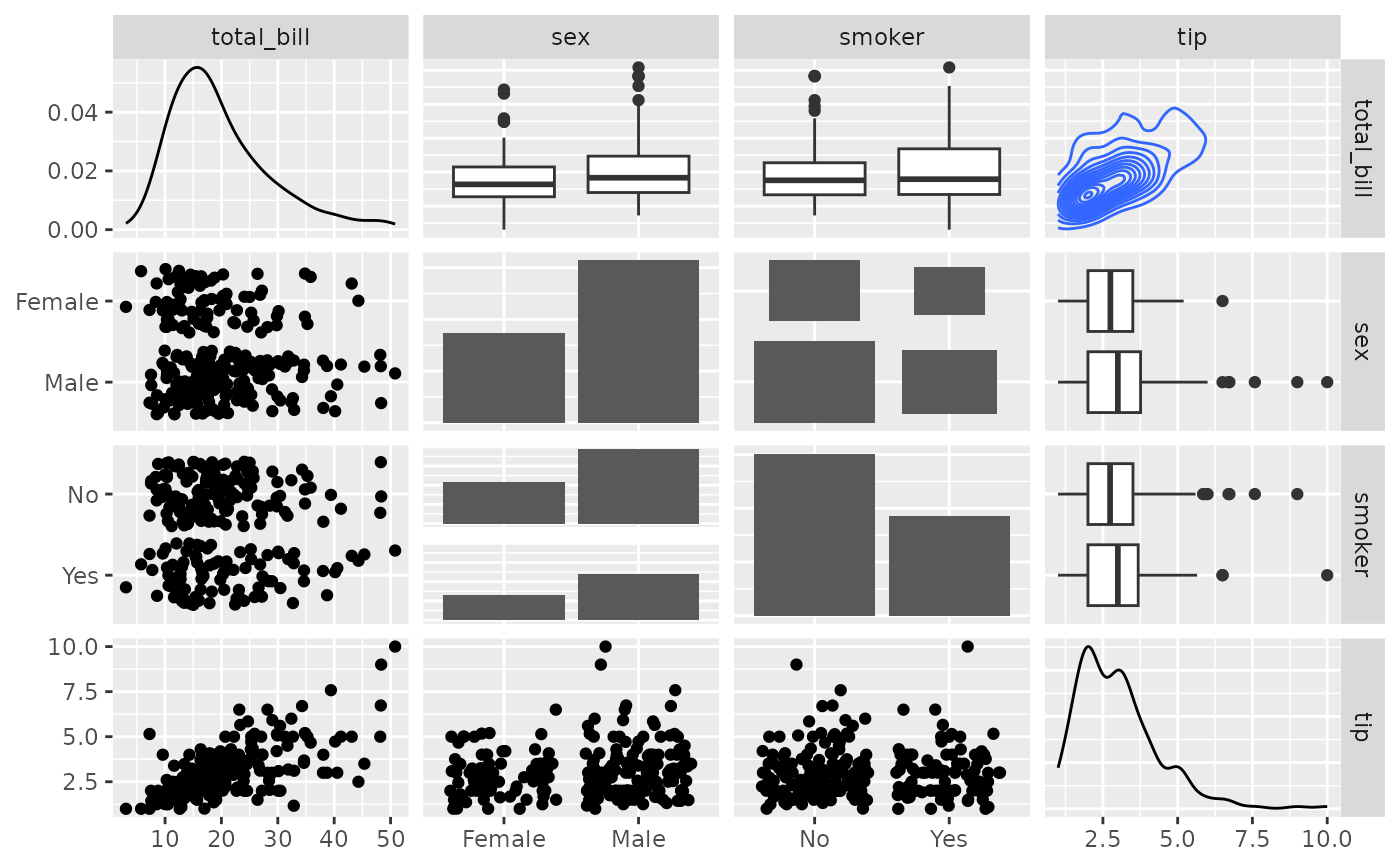# Supply Raw Functions (may be user defined functions!)
pm <- ggpairs(
tips[, c(1, 3, 4, 2)],
upper = list(continuous = ggally_density, combo = ggally_box_no_facet),
lower = list(continuous = ggally_points, combo = ggally_dot_no_facet)
)
p_(pm)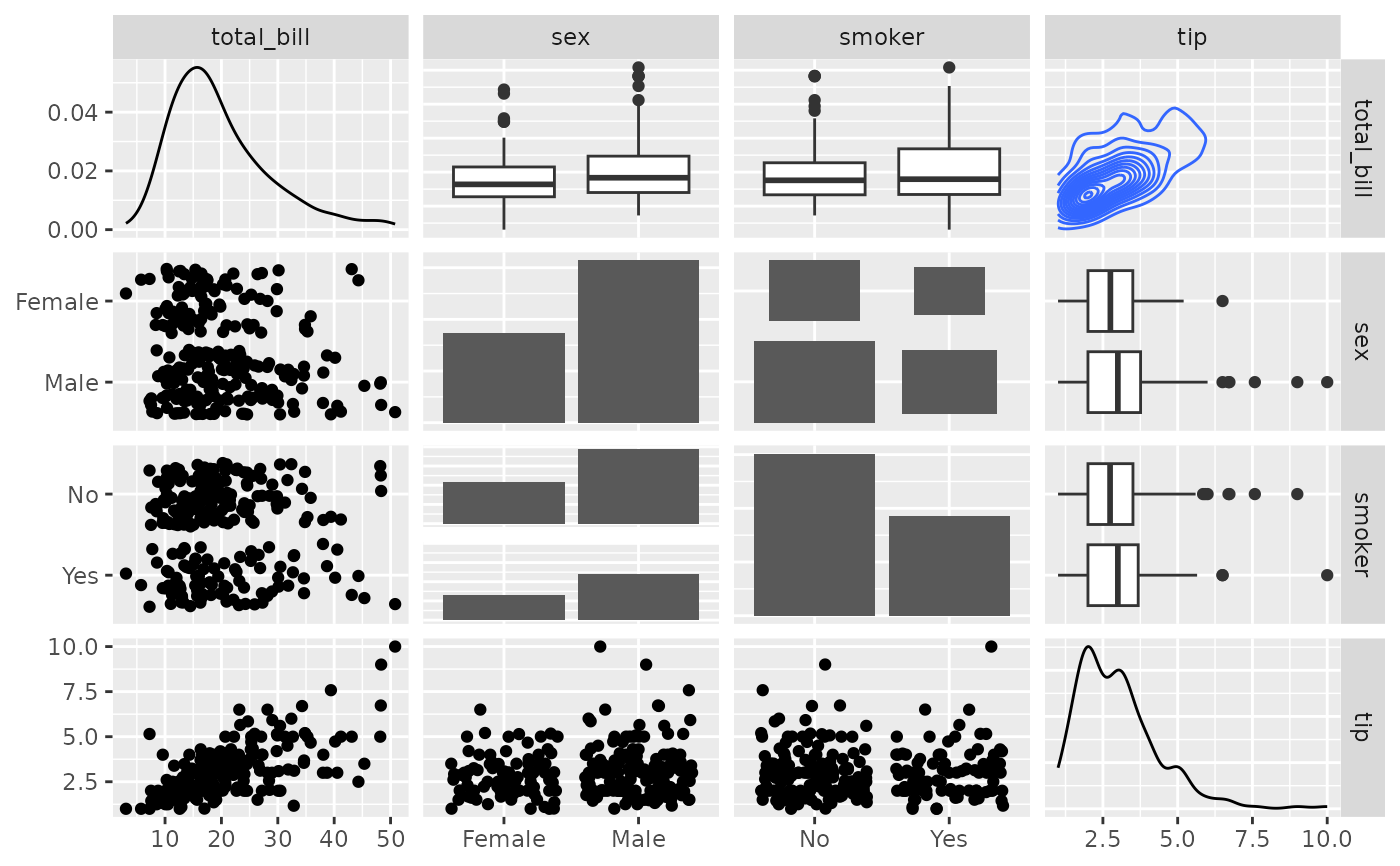# Use sample of the diamonds data
data(diamonds, package = "ggplot2")
diamonds.samp <- diamonds[sample(1:dim(diamonds), 1000), ]

# Different aesthetics for different plot sections and plot types
pm <- ggpairs(
diamonds.samp[, 1:5],
mapping = ggplot2::aes(color = cut),
upper = list(continuous = wrap("density", alpha = 0.5), combo = "box_no_facet"),
lower = list(continuous = wrap("points", alpha = 0.3), combo = wrap("dot_no_facet", alpha = 0.4)),
title = "Diamonds"
)
p_(pm)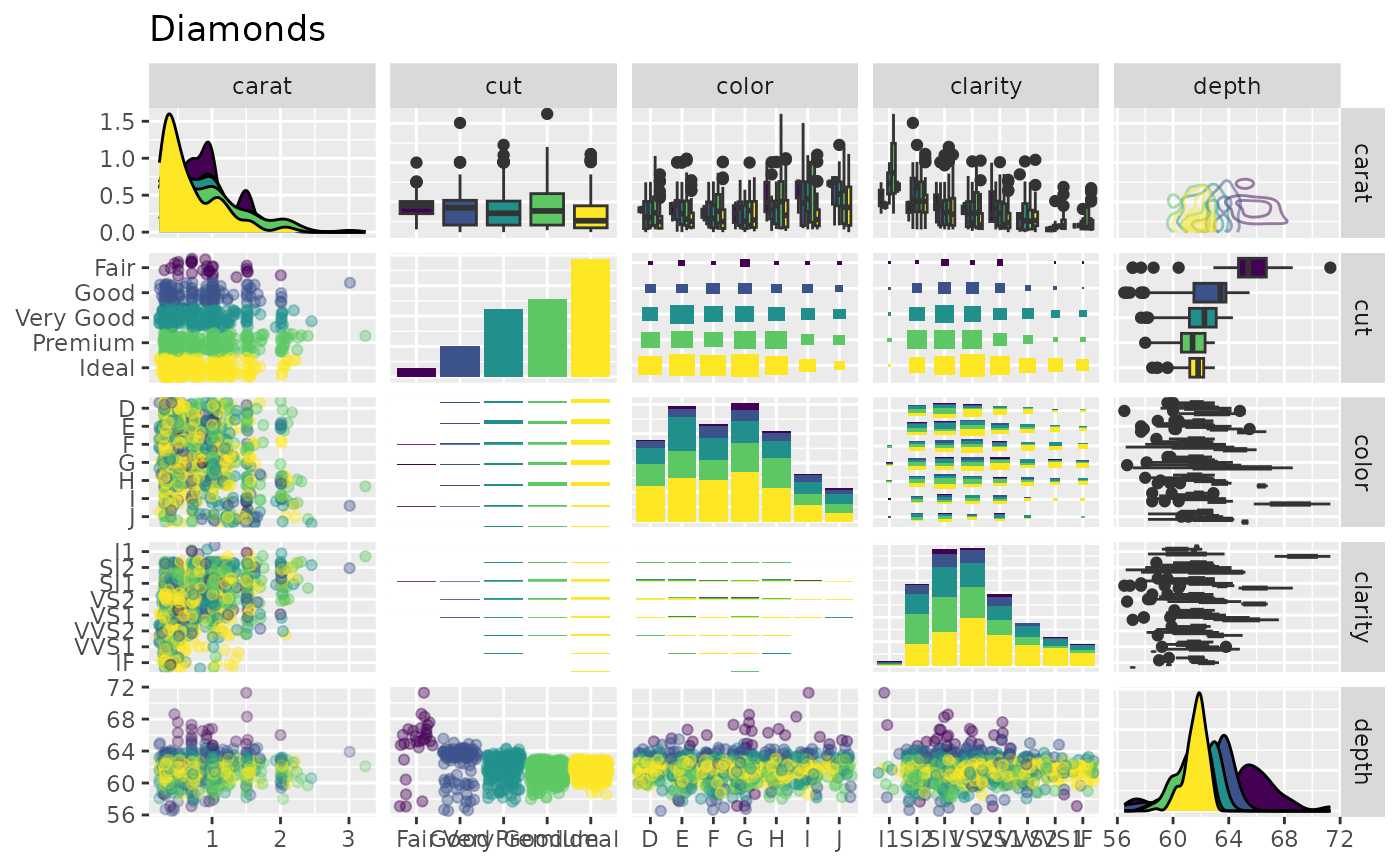## Axis Label Variations
# Only Variable Labels on the diagonal (no axis labels)
pm <- ggpairs(tips[, 1:3], axisLabels = "internal")
p_(pm)
#> stat_bin() using bins = 30. Pick better value with binwidth.
#> stat_bin() using bins = 30. Pick better value with binwidth.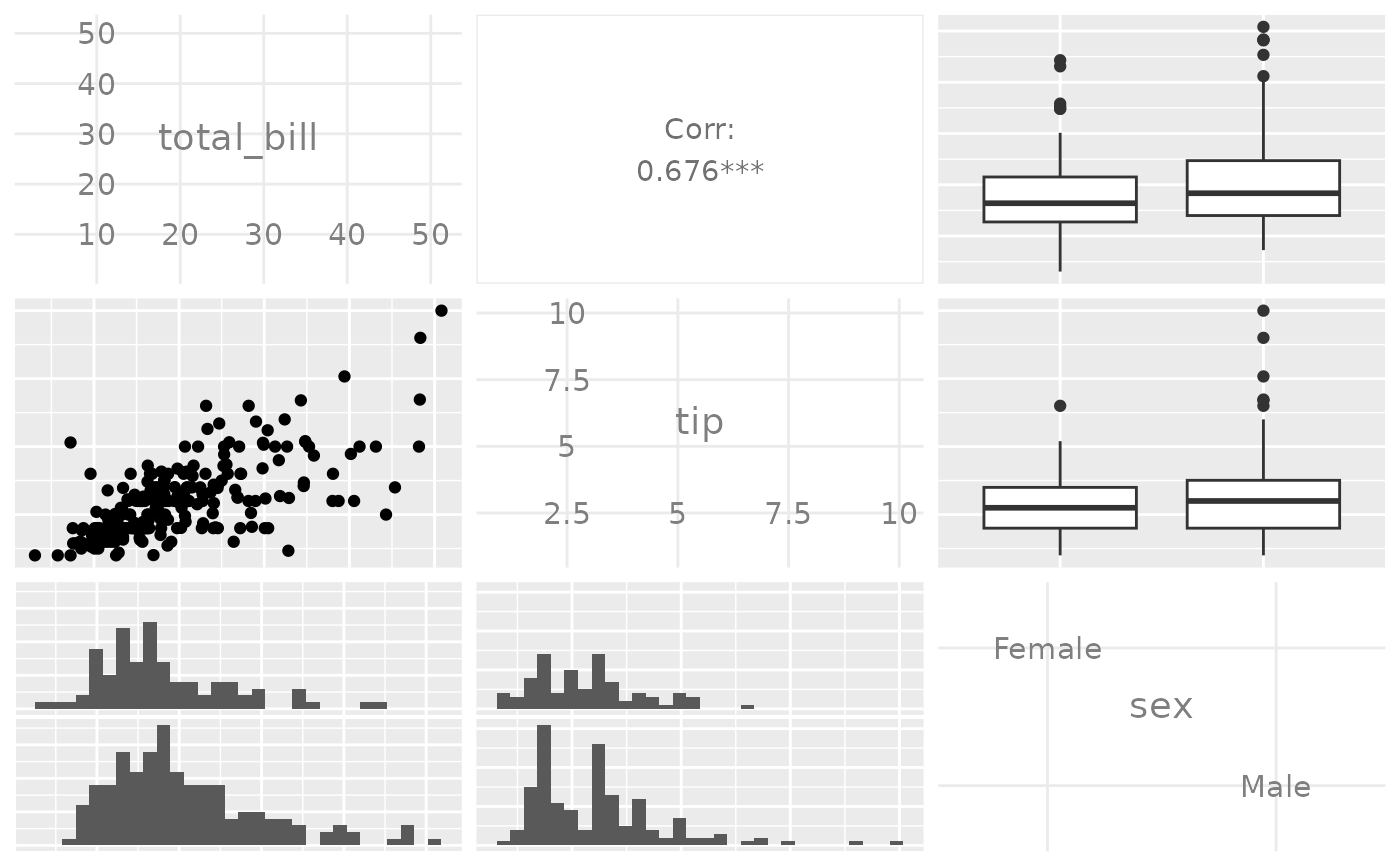# Only Variable Labels on the outside (no axis labels)
pm <- ggpairs(tips[, 1:3], axisLabels = "none")
p_(pm)
#> stat_bin() using bins = 30. Pick better value with binwidth.
#> stat_bin() using bins = 30. Pick better value with binwidth.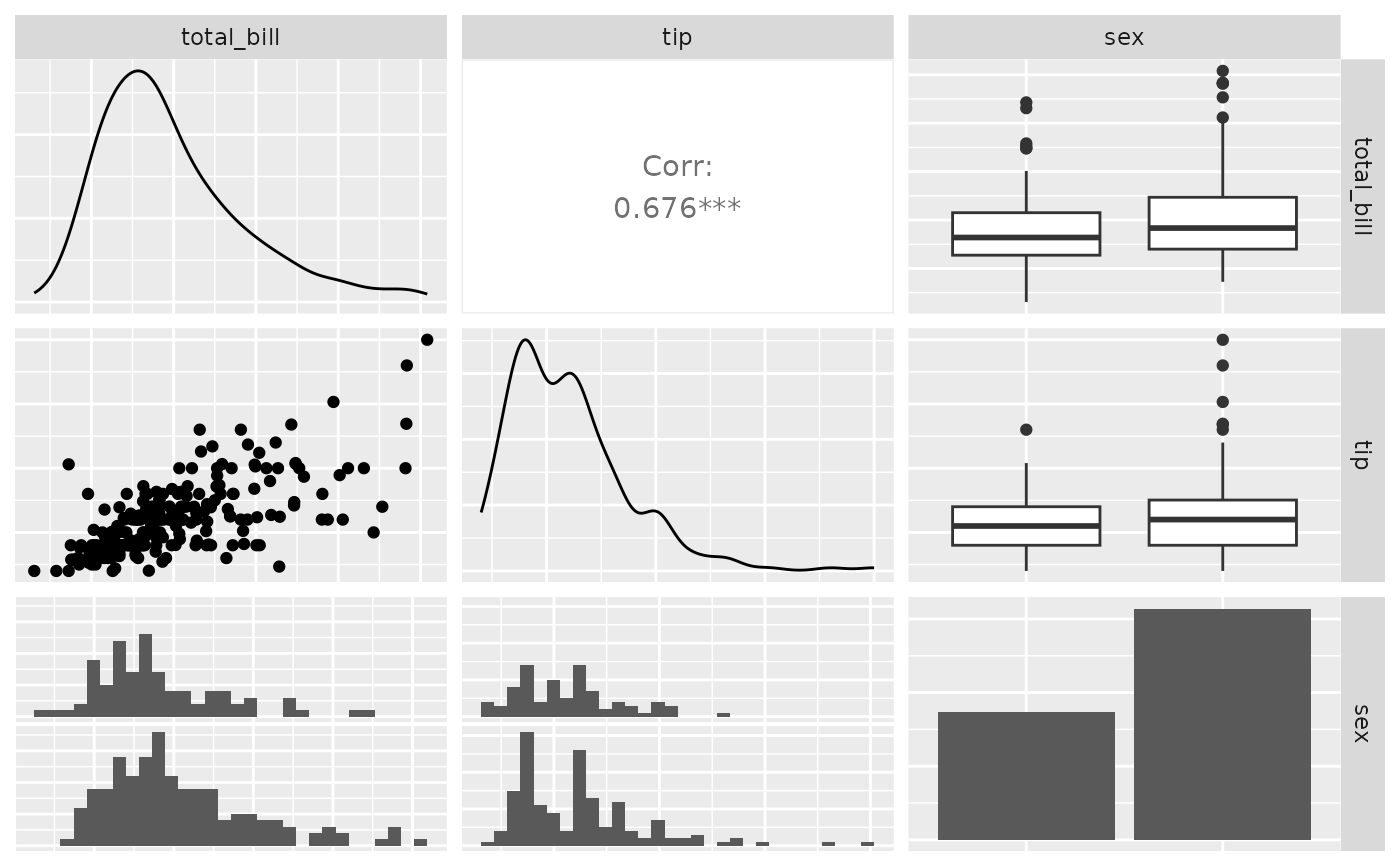## Facet Label Variations
#  Default:
df_x <- rnorm(100)
df_y <- df_x + rnorm(100, 0, 0.1)
df <- data.frame(x = df_x, y = df_y, c = sqrt(df_x^2 + df_y^2))
pm <- ggpairs(
df,
columnLabels = c("alpha[foo]", "alpha[bar]", "sqrt(alpha[foo]^2 + alpha[bar]^2)")
)
p_(pm)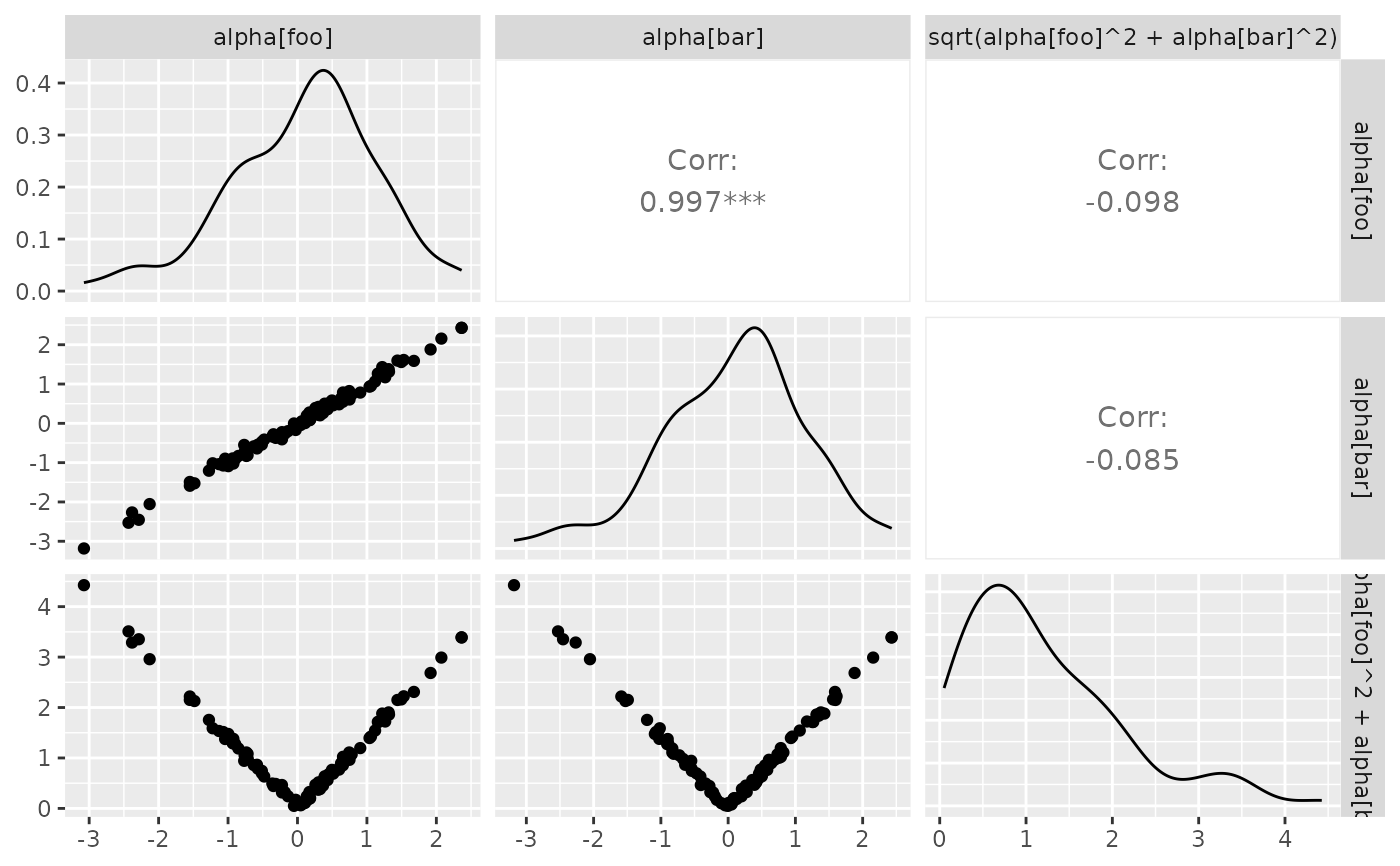#  Parsed labels:
pm <- ggpairs(
df,
columnLabels = c("alpha[foo]", "alpha[bar]", "sqrt(alpha[foo]^2 + alpha[bar]^2)"),
labeller = "label_parsed"
)
p_(pm)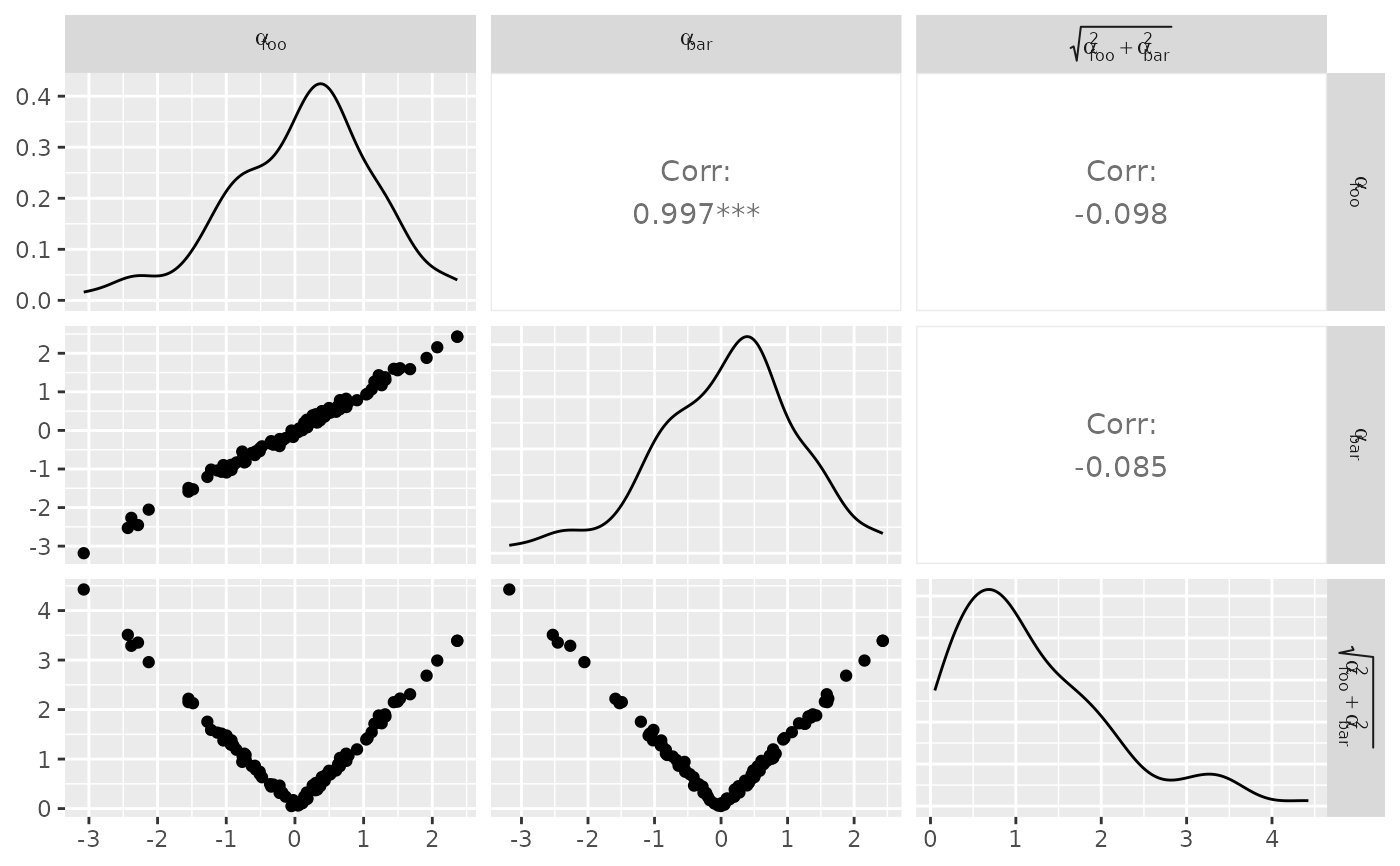## Plot Insertion Example
custom_car <- ggpairs(mtcars[, c("mpg", "wt", "cyl")], upper = "blank", title = "Custom Example")
# ggplot example taken from example(geom_text)
plot <- ggplot2::ggplot(mtcars, ggplot2::aes(x = wt, y = mpg, label = rownames(mtcars)))
plot <- plot +
ggplot2::geom_text(ggplot2::aes(colour = factor(cyl)), size = 3) +
ggplot2::scale_colour_discrete(l = 40)
custom_car[1, 2] <- plot
personal_plot <- ggally_text(
"ggpairs allows you\nto put in your\nown plot.\nLike that one.\n <---"
)
custom_car[1, 3] <- personal_plot
p_(custom_car)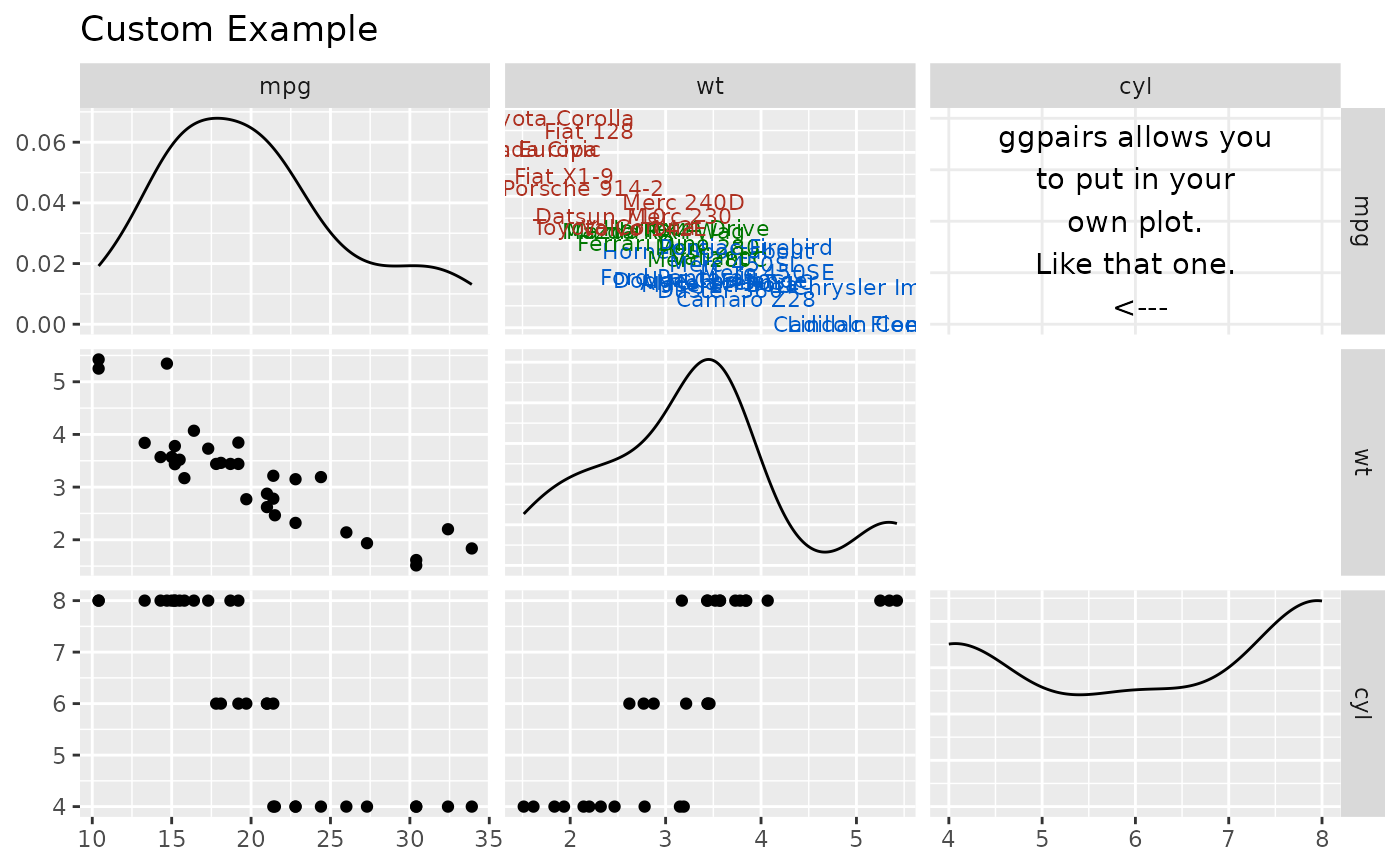## Remove binwidth warning from ggplot2
# displays warning about picking a better binwidth
pm <- ggpairs(tips, 2:3)
p_(pm)
#> stat_bin() using bins = 30. Pick better value with binwidth.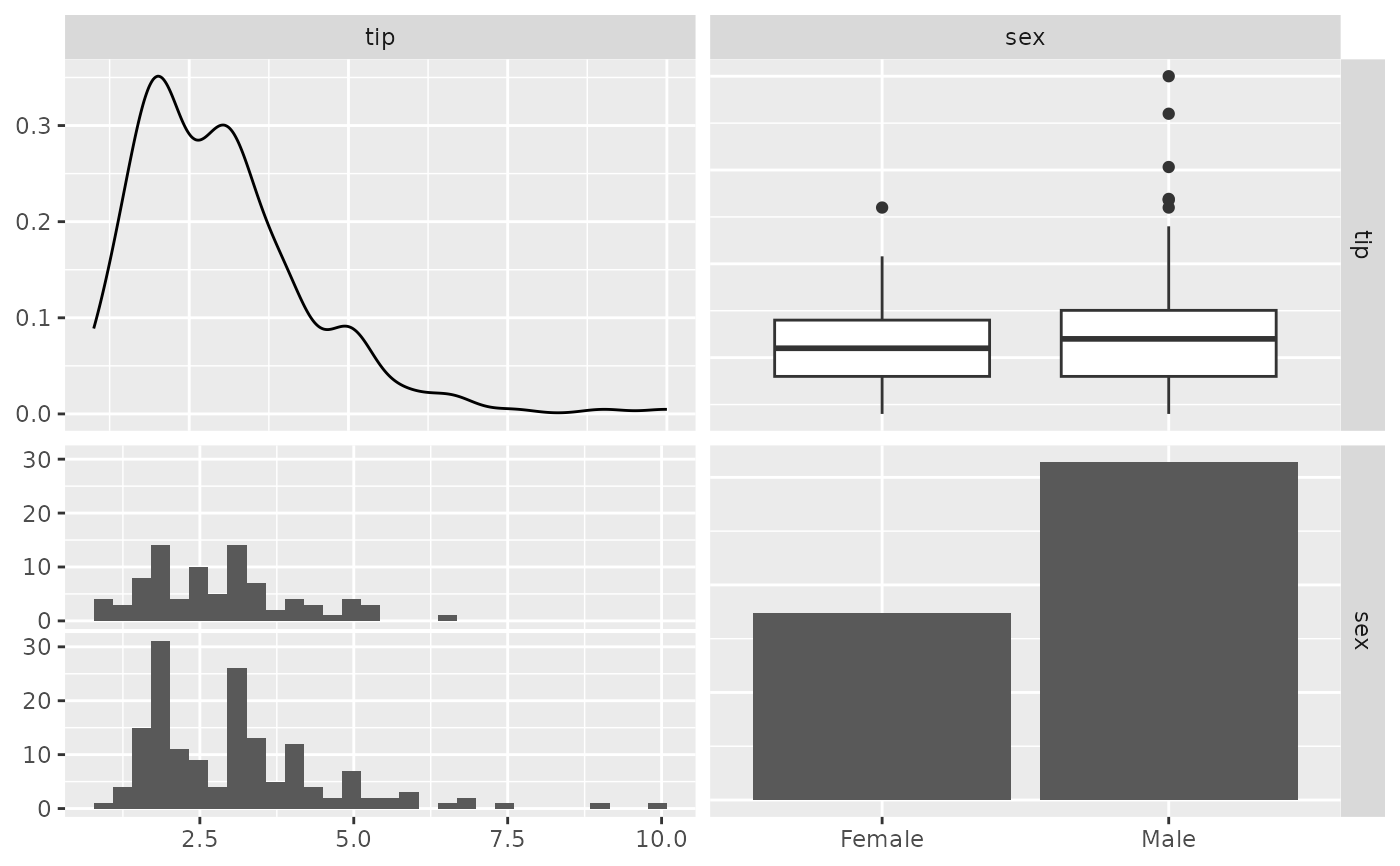# no warning displayed
pm <- ggpairs(tips, 2:3, lower = list(combo = wrap("facethist", binwidth = 0.5)))
p_(pm)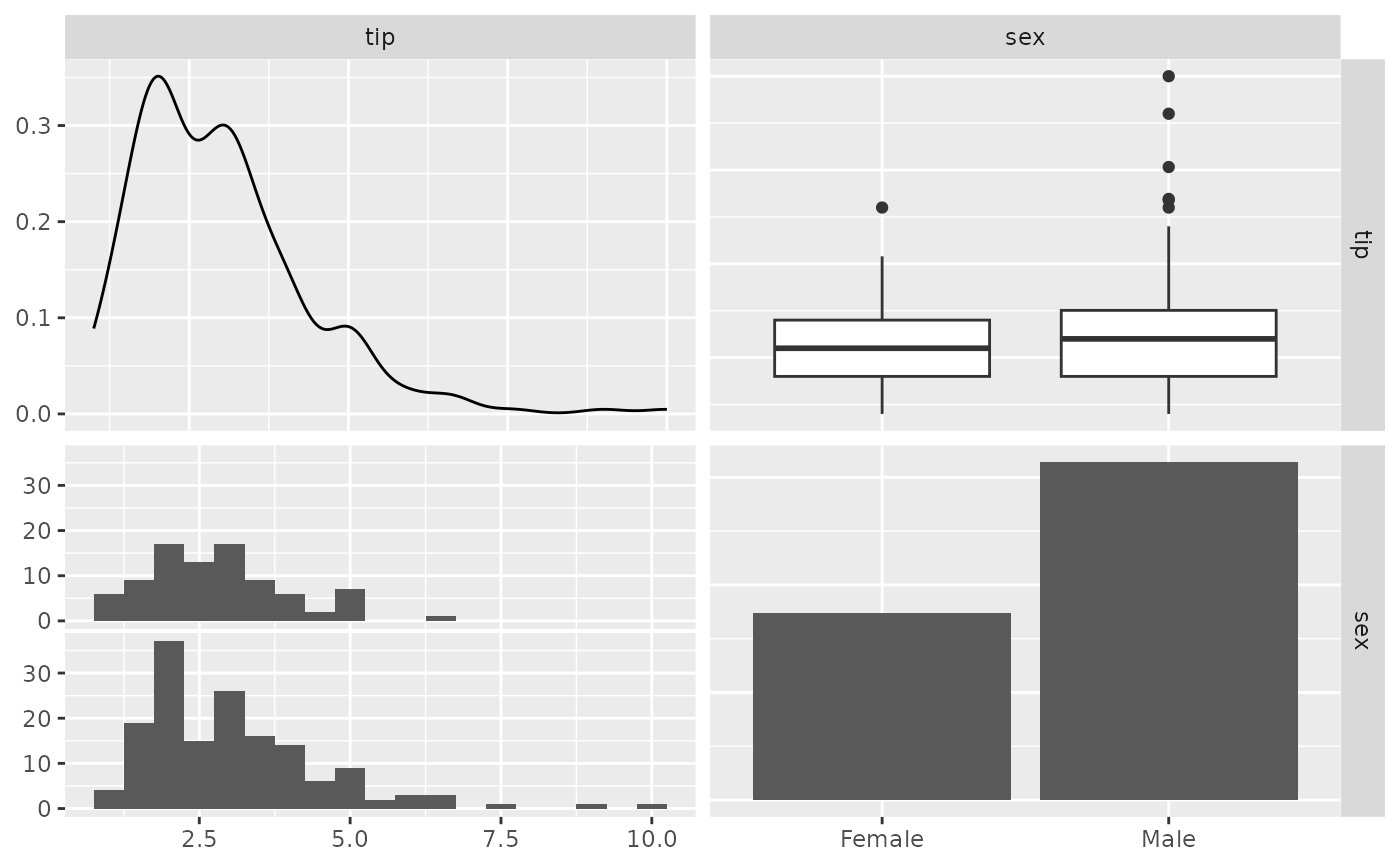# no warning displayed with user supplied function
pm <- ggpairs(tips, 2:3, lower = list(combo = wrap(ggally_facethist, binwidth = 0.5)))
p_(pm)## Remove panel grid lines from correlation plots
pm <- ggpairs(
flea, columns = 2:4,
upper = list(continuous = wrap(ggally_cor, displayGrid = FALSE))
)
p_(pm)
#> Warning: displayGrid is deprecated. Please use display_grid
#> Warning: displayGrid is deprecated. Please use display_grid
#> Warning: displayGrid is deprecated. Please use display_grid## Custom with/height of subplots
pm <- ggpairs(tips, columns = c(2, 3, 5))
p_(pm)
#> stat_bin() using bins = 30. Pick better value with binwidth.
#> stat_bin() using bins = 30. Pick better value with binwidth.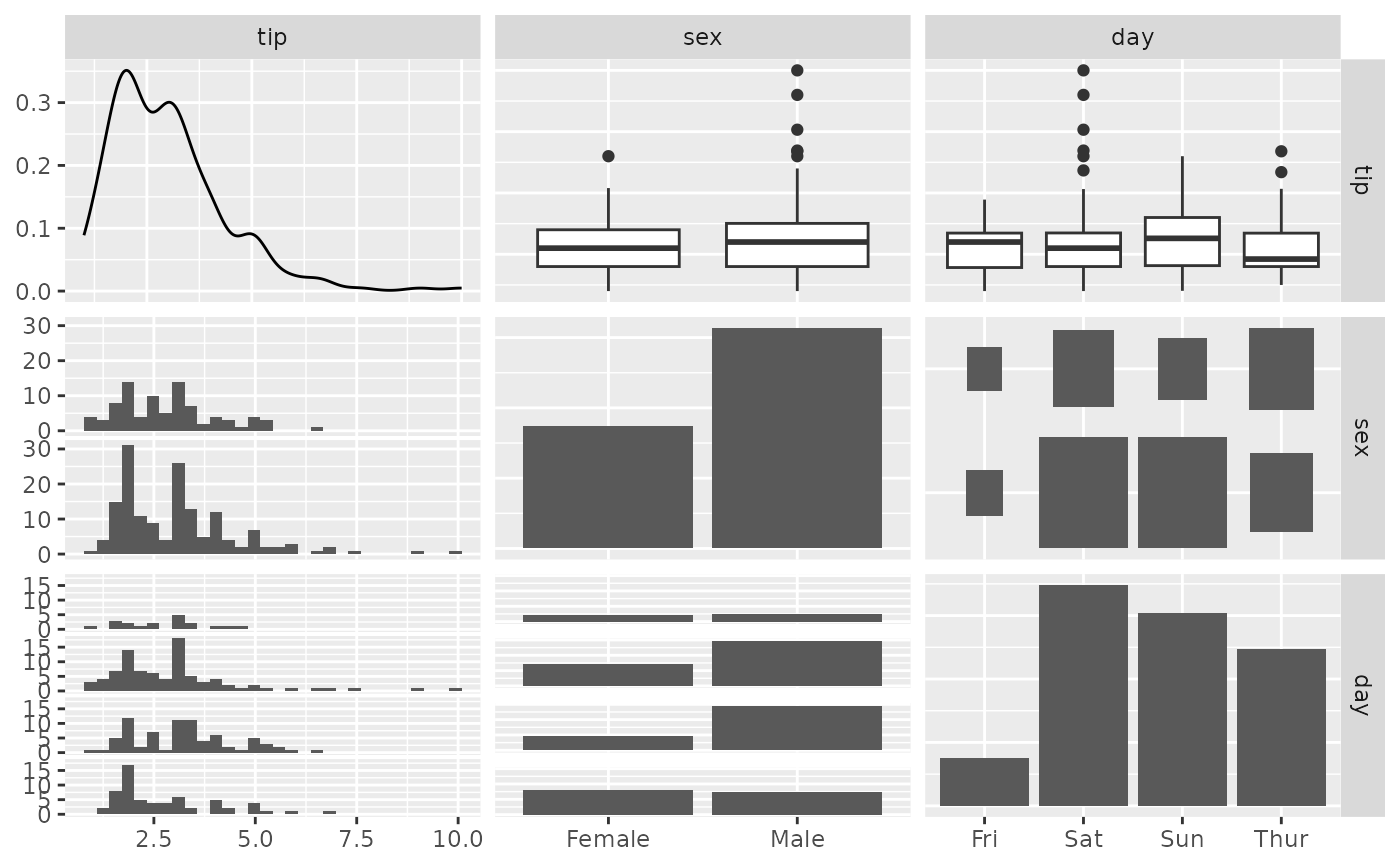pm <- ggpairs(tips, columns = c(2, 3, 5), proportions = "auto")
p_(pm)
#> stat_bin() using bins = 30. Pick better value with binwidth.
#> stat_bin() using bins = 30. Pick better value with binwidth.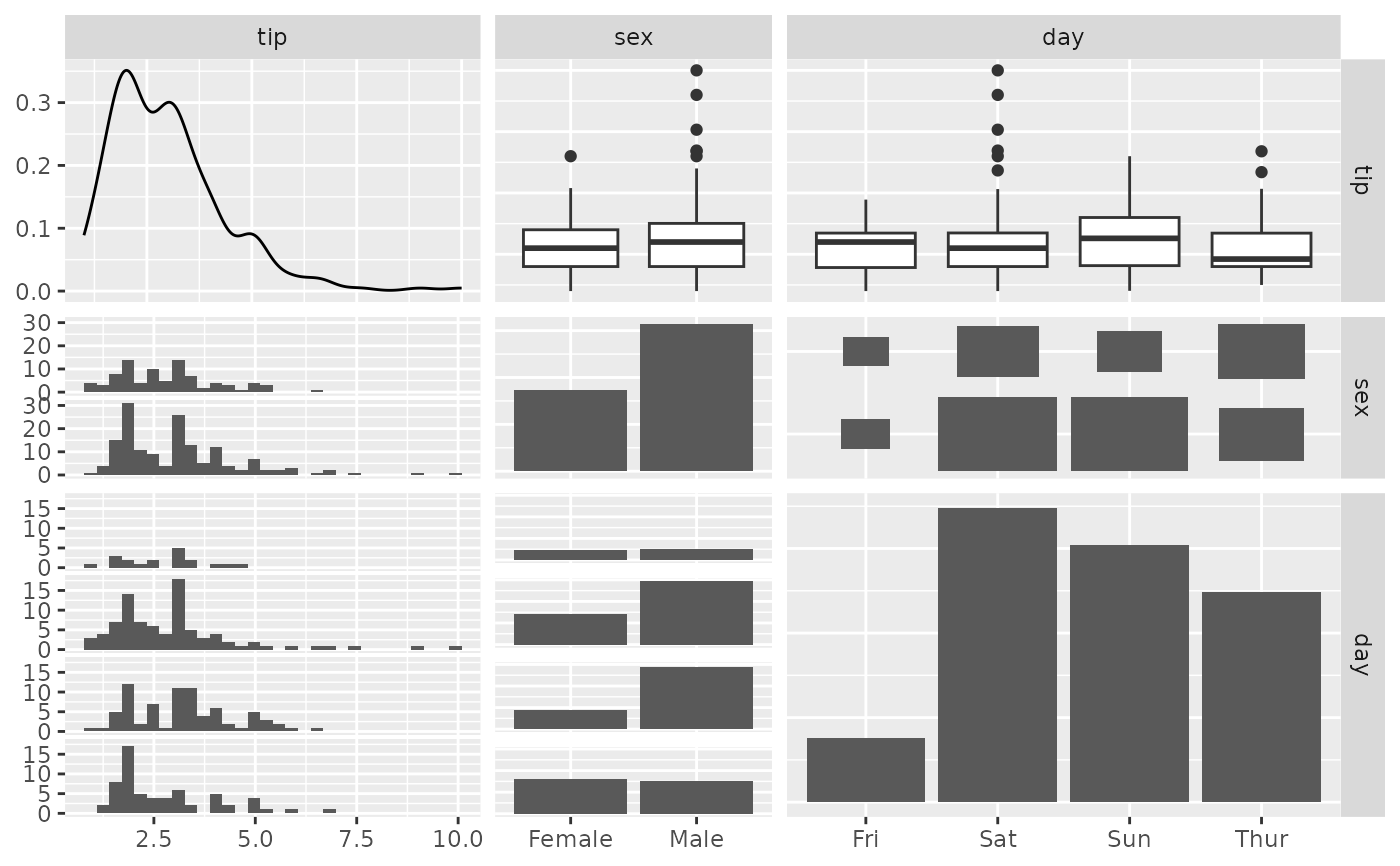pm <- ggpairs(tips, columns = c(2, 3, 5), proportions = c(1, 3, 2))
p_(pm)
#> stat_bin() using bins = 30. Pick better value with binwidth.
#> stat_bin() using bins = 30. Pick better value with binwidth.